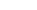•WE PROVIDEHELP WITH
R PROJECTS
We help with
Descriptive Statistics
Hypothesis Test
Factorial ANOVA
and all R in general
starts at \$35/hour.

# R Homework Help

## R Projects Topics

• Survey Analysis
• Design of surveys
• Reports - Crosstabs
• Scales - Reliability - Cronbach's Alpha
• Factor Analysis - Principal Components
• Validity
• Questionnaire Analysis using R
• Discriminant Analysis

• Data Analysis, Sampling and Charts
• Sampling Methods: Random, Stratified, Cluster, etc.
• Histograms
• Stem and Leaf
• Box-Plot
• Normality Tests (Anderson-Darling, Kolmogorov)

• Measures of Central Tendency and Dispersion
• Mean
• Standard Deviation
• Variance
• Standard Error of the Mean
• Range
• Skewness
• Coefficient of variation

• Regression Analysis
• Coefficient of Correlation
• Coefficient of Determination
• Least Squares Method
• Multiple Linear Regression
• Multivariate Statistical Analysis
• Logistic Regression in R

• Times Series
• Control Charts
• Autocorrelation
• Seasonal Indexes
• Trends, cycles
• Forecasting

• Probability Distributions
• Binomial Distribution
• Poisson Distribution
• Exponential Distribution
• Standard Normal Distribution

• Hypothesis Testing
• Z-test, two independent samples
• t-test, two independent samples, paired samples
• F-test
• Non-parametric Chi Square test
• One Way and Two way ANOVA
• Factorial ANOVA
• ANCOVA and MANOVA in R
• Levene's Test
• Crosstabs
• Chi Square Tests
• Non-Parametric Tests

## Claim Your Free Solved Stats Problems!

* indicates required
Email Format

## Our Specialties

#### R Syntax

We can help you with conducting all of sorts of Statistical Tests using R, with syntax commands, and provide you with accurate interpretation of the outputs, along with a write up of the results, including all the necessary tables and charts.

#### Regression Analysis

We can help with all kinds of homework involving Simple Linear Regression, Multiple Linear Regression, Hierarchical Regression, Logistic Analysis, Discriminant Analysis, by using any of the most popular statistical software packages

#### Descriptive Statistics

We have helped thousands of customers with all kinds of Stats assignments involving Descriptive Statistics, such the calculation of measures of central tendency (Mean, Median, Mode), measures of dispersion (Standard Deviation, Variance, Range, Interquartile Range), quartiles, and with the description of distributional properties (normal distribution, etc.)

#### Hypothesis Testing

We can help you with z-tests, t-tests, ANOVA, Chi-Square test, and the parametric tests you may need help with, by using R

#### Advanced Statistical Analyses with R

We don't only do Basic Stats, we can also handle all kind of Advanced statistics projects, with any statistical software aside from R

#### We provide help with ALL Math Subjects

Aside from Stats, we also do Calculus, Algebra, Operations Management, Quantitative Methods, Decision Analysis, you name it

#### We are a partner you can trust

We have been online more than 10 years and we have worked successfully with thousand of satisfied customers